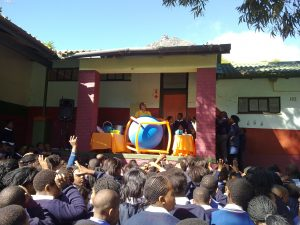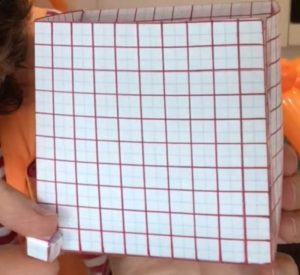Watch the video about the Metre Cube and Scale.

How many children can fit inside a metre cube (1 metre by 1 metre by 1 metre)? How many adults?

How many cubic centimetres (1 centimetre by 1 centimetre by 1 centimetre) can fit inside a metre cube?These cubes are made from centimetre squared paper and measure 10 centimetres by 10 centimetres by 10 centimetres and 1 centimetre by 1 centimetre by 1 centimetre. How many of the smaller 1 cubic centimetre cubes can you pack into the bigger cube?

1. What is the edge length of the 10 by 10 by 10 cube?
2. You can see how many square centimetres there are on each face. What is the surface area of the whole 10 by 10 by 10 cube?
3. What is it’s volume?
4. What is the linear scale factor from the 1 by 1 by 1 cube to the 10 by 10 by 10 cube?
5. What is the area scale factor?
6. What is the volume scale factor?
7. How many of the smaller 1 by 1 by 1 (1 cubic centimetre) cubes can you pack into the 10 by 10 by 10 cube?
8. How many of the 1 by 1 by 1 cubes can you pack into a metre cube?
9. What is the surface area of the metre cube?
10. What is the linear scale factor comparing the metre cube (100 by 100 by 100) to the 10 by 10 by 10 cube and to the 1 by 1 by 1 cube?
11. What is the area scale factor comparing the metre cube (100 by 100 by 100) to the 10 by 10 by 10 cube and to the 1 by 1 by 1 cube?
12. What is the volume scale factor comparing the metre cube (100 by 100 by 100) to the 10 by 10 by 10 cube and to the 1 by 1 by 1 cube?